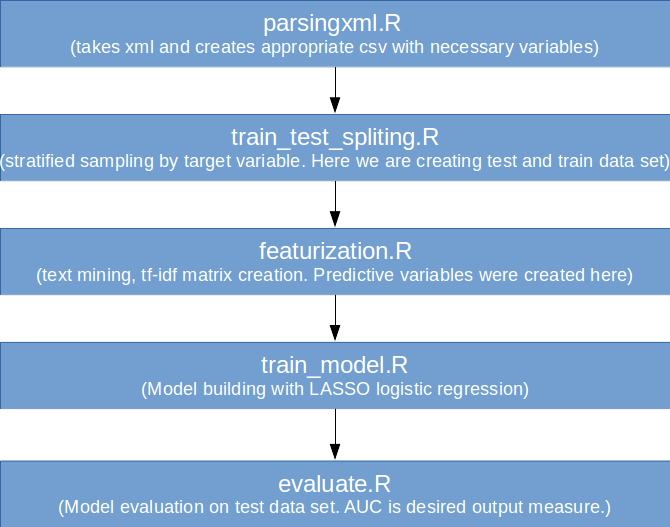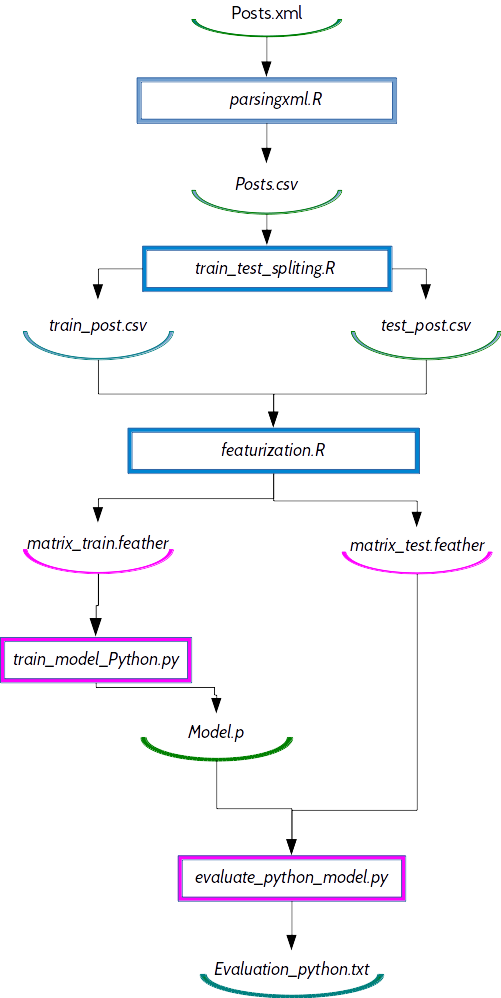# Best practices of orchestrating Python and R code in ML projects

Instead of arguing about Python vs R I will examine the best practices of integrating both languages in one data science project.

By Marija Ilic, Data scientist at Zagrebacka banka.Image was taken from this page

Today, data scientists are generally divided among two languages — some prefer R, some prefer Python. I will not try to explain in this article which one is better. Instead of that I will try to find an answer to a question: “What is the best way to integrate both languages in one data science project? What are the best practices?”. Beside git and shell scripting additional tools are developed to facilitate the development of predictive model in a multi-language environments. For fast data exchange between R and Python let’s use binary data file format Feather. Another language agnostic tool DVC can make the research reproducible — let’s use DVC to orchestrate R and Python code instead of a regular shell scripts.

### Machine learning with R and Python

Both R and Python are having powerful libraries/packages used for predictive modeling. Usually algorithms used for classification or regression are implemented in both languages and some scientist are using R while some of them preferring Python. In an example that was explained in previous tutorialtarget variable was binary output and logistic regression was used as a training algorithm. One of the algorithms that could also be used for prediction is a popular Random Forest algorithm which is implemented in both programming languages. Because of performances it was decided that Random Forest classifier should be implemented in Python (it shows better performances than random forest package in R).

### R example used for DVC demo

We will use the same example from previous blog story, add some Python codes and explain how Feather and DVC can simplify the development process in this combined environment.

Let’s recall briefly the R codes from previous tutorial:R jobs

Input data are Stackoverflow posts — an XML file. Predictive variables are created from text posts — relative importance tf-idf of words among all available posts is calculated. With tf-idf matrices target is predicted and lasso logistic regression for predicting binary output is used. AUC is calculated on the test set and AUC metric is used on evaluation.

Instead of using logistic regression in R we will write Python jobs in which we will try to use random forest as training model. Train_model.R and evaluate.R will be replaced with appropriate Python jobs.

R codes can be seen here.

Code for train_model_Python.py is presented below:

```import numpy as np
from sklearn.ensemble import RandomForestClassifier
import sys
try: import cPickle as pickle   # python2
except: import pickle           # python3
from scipy import sparse
import feather as ft

if len(sys.argv) != 4:
sys.stderr.write('Arguments error. Usage:\n')
sys.stderr.write('\tpython train_model.py
INPUT_MATRIX_FILE SEED OUTPUT_MODEL_FILE\n')
sys.exit(1)

input = sys.argv
seed = int(sys.argv)
output = sys.argv

labels = df.loc[:,'label']
x = df.loc[:, df.columns != 'label']

clf = RandomForestClassifier(n_estimators=100, n_jobs=2, random_state=seed)
clf.fit(x, labels.ix[:,0])

with open(output, 'wb') as fd:
pickle.dump(clf, fd)
```

Also here we are adding code for evaluation_python_model.py:

```from sklearn.metrics import precision_recall_curve
import sys
import sklearn.metrics as metrics
from scipy import sparse
try: import cPickle as pickle   # python2
except: import pickle           # python3
import feather as ft

if len(sys.argv) != 4:
sys.stderr.write('Arguments error. Usage:\n')
sys.stderr.write('\tpython metrics.py
MODEL_FILE TEST_MATRIX METRICS_FILE\n')
sys.exit(1)

model_file = sys.argv
test_matrix_file = sys.argv
metrics_file = sys.argv

with open(model_file, 'rb') as fd:

labels = df.loc[:,'label']
x = df.loc[:, df.columns != 'label']
predictions_by_class = model.predict_proba(x)
predictions = predictions_by_class[:,1]

precision, recall, thresholds = precision_recall_curve(
labels.ix[:,0], predictions)

auc = metrics.auc(recall, precision)
#print('AUC={}'.format(metrics.auc(recall, precision)))
with open(metrics_file, 'w') as fd:
fd.write('AUC: {:4f}\n'.format(auc))
```

Let’s download necessary R and Python codes from above (clone the Githubrepository):

```mkdir R_DVC_GITHUB_CODE
cd R_DVC_GITHUB_CODE```
`git clone https://github.com/Zoldin/R_AND_DVC`

Our dependency graph of this data science project look like this:Now lets see how it is possible to speed up and simplify process flow with Feather API and data version control reproducibility.